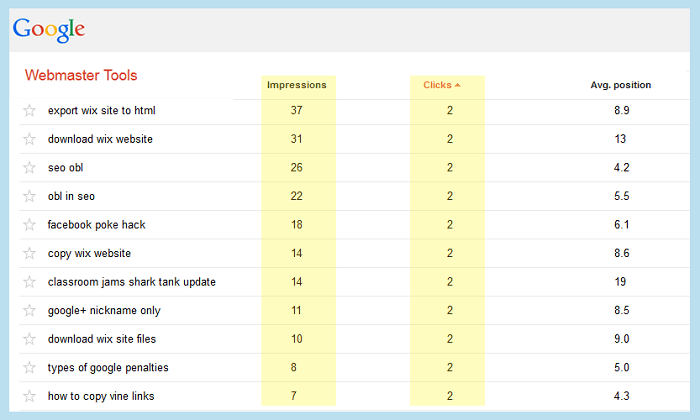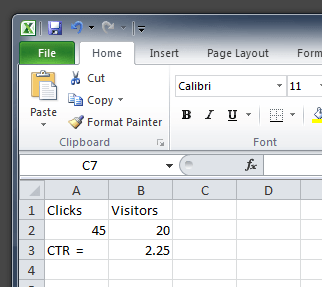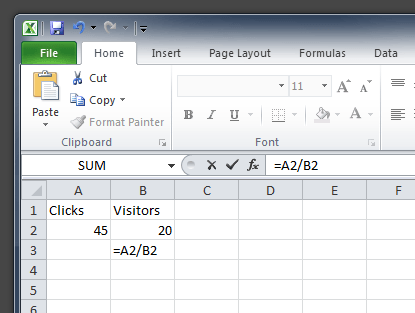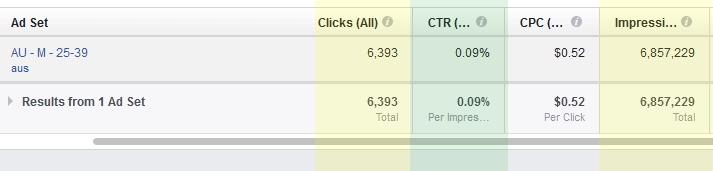This article will outline exactly how to calculate the click through rate or CTR of a website using Microsoft Excel or using a simple calculator. We’ll also talk about conversion rate, and how CTR varies in SEO, PPC, and Facebook marketing.

Note: I wrote this article originally in 2011 when I was just starting out with blogging. It has been rewritten for simplification purposes and to keep things up to date. Let’s start off by saying that there are many tools that can calculate these formulas (CTR and conversion rate) that have already been created.  SEO’s use Microsoft Excel because it is quick and easy, and integrates very well with a lot of other SEO tools. A lot of people in the SEO and marketing industry like to export their data into Excel for further crunching, so this formula can be very useful when working with other types of numbers. Anyhow, this is somewhat of an intermediate topic but we will keep it really simple.  We assume that you know what CTR, CPC, and what a conversion means. We also assume that you have a very basic understanding of Microsoft Excel before using this.  I’m going to go over 2 basic Excel calculations here today that will help you get more bang for your buck with your data.

## CTR can mean different things in SEO and PPC

In SEO, click-through rate (CTR) refers to the % of clicks you receive divided by the number of impressions you receive in the search engines. This can only be calculated by taking the number of impressions in webmaster tools and dividing by the number of clicks. Number of clicks can be taken from your analytics or via Google Webmaster Tools however be warned Google Webmaster Tools click data isn’t always accurate.In internet marketing, affiliate marketing or internet advertising click-through rate (CTR) is calculated differently than the way SEO click through rate is. In affiliate marketing / advertising you take the amount of clicks you received and divide them by the number of impressions you have. Pretty simple.

## Calculating Click Through Rate or CTR in Microsoft Excel

CTR is calculated the same way for SEO or affiliate marketing. No matter what the case is, the formula is this:

```CTR or Click Through Rate in organic SEO = Clicks / Impressions * 100
CTR or Click Through Rate in internet marketing = Clicks / Impressions * 100
```

Just remember to always use the same date ranges for clicks and impressions. For example don’t calculate impressions for the whole year divided by clicks for this week. That’ll leave you with some whack data. Now let’s take a look at how to calculate this in Microsoft Excel. Most webmasters keep track of their statistics in Excel to track a number of different metrics.  It can be very time consuming to calculate these formulas over and over again, and you also don’t want to introduce human error into the equation.  Here is how you would calculate CTR in Microsoft Excel: Identify which cell you want to display the Click Through Rate. Within that cell, type:

``` "=CELL_WITH_CLICKS/CELL_WITH_VISITORS"
or in the case of the example below it would be:
=A2/B2```As you can see, this is pretty straightforward.  You are basically dividing one cell into another, and displaying the output into another one.  Give it a try with a blank Excel Worksheet if you want.

There is actually one more step in Excel. You can either edit the formula to be (CELL1/Cell2*100) OR just modify the cell formatting to be a “percentage.” You can also play with the decimals if you want more or less accuracy. The Excel CTR calculator below uses the *100 method.

## What is Facebook Click Through Rate (CTR)?

You calculate CTR in Facebook using the same method described above. The good thing about Facebook CTR is that they already calculate it for you. Just look at any campaign and adjust your columns appropriately:Note: the above image is an example of a really bad click through rate on Facebook :)

In the above example, the CTR is about .09% or almost .1%. This is calculated by taking the number of impressions (about 6 million) and diving them by the number of clicks (about 6000) then multiplying that by 100.

## Calculating Conversion Rate in Excel

Next is one that is a little bit more complicated concept yet easy formula, and that is calculating conversion rate.  When calculating the conversion rate you should have the following information available:

• how many sales or conversions you’ve made
• amount of clicks or visitors

Now sales or conversions can be any number of metrics such as:

• items you’ve sold on an eCommerce website
• app installs
• service requests
• or even email addresses collected

The formula for calculating conversion rate is as follows:

`number of conversions  / traffic  * 100`

So lets say I have 350 signups If you’re looking in Excel, your data will look something like this: =B1/B2*100 or you can ditch the multiplying by 100 and just change the cell format to a  “percentage” type. Using this formula, Excel will automatically calculate the conversion rate.